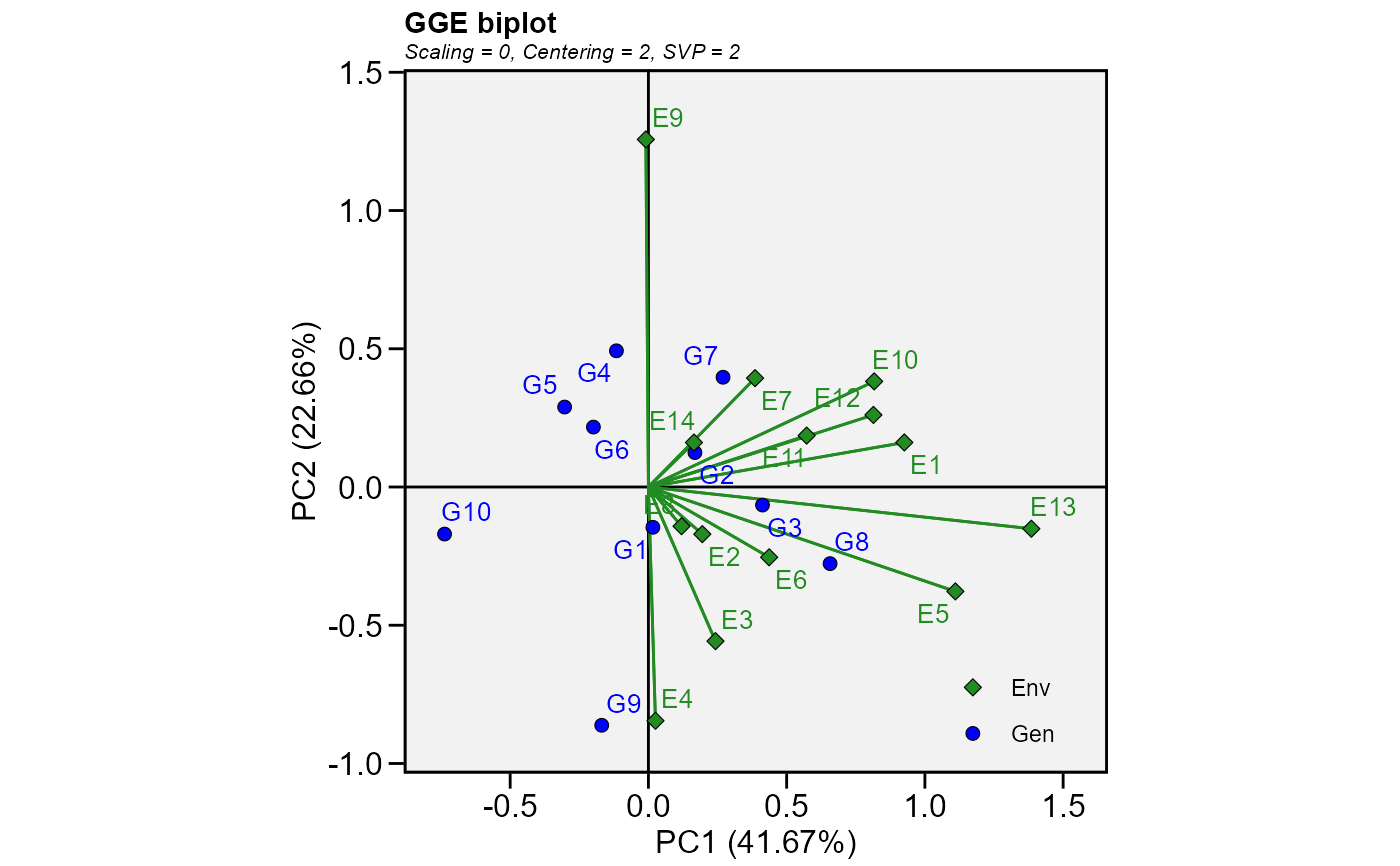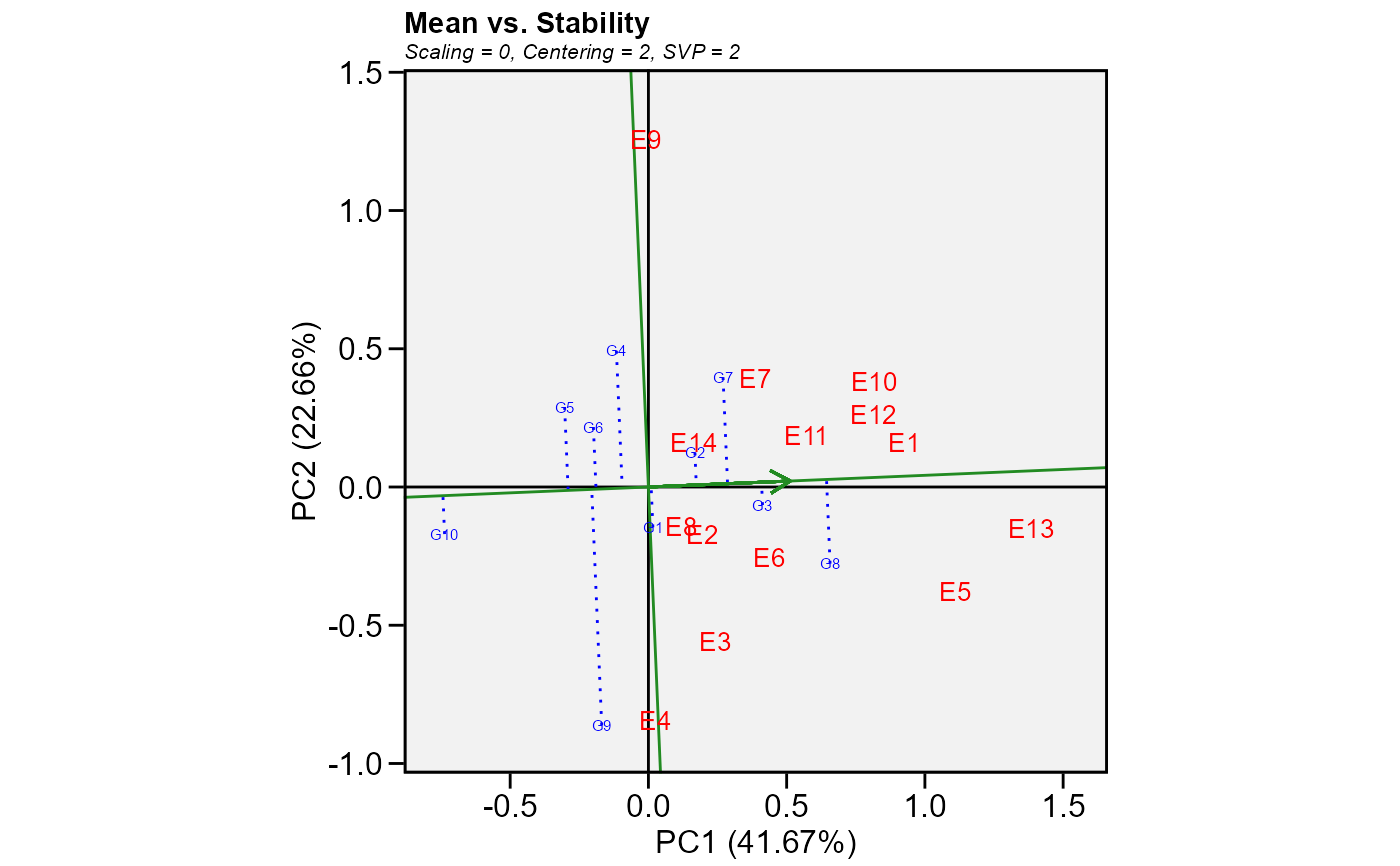Produces a ggplot2-based GGE-GT-GYT biplot based on a model fitted with the functions gge(), gtb(), and gytb().

# S3 method for gge
plot(
x,
var = 1,
type = 1,
sel_env = NA,
sel_gen = NA,
sel_gen1 = NA,
sel_gen2 = NA,
shape.gen = 21,
shape.env = 23,
line.type.gen = "dotted",
size.shape = 2.2,
size.shape.win = 3.2,
size.stroke = 0.3,
col.stroke = "black",
col.gen = "blue",
col.env = "forestgreen",
col.line = "forestgreen",
col.alpha = 1,
col.circle = "gray",
col.alpha.circle = 0.5,
leg.lab = NULL,
size.text.gen = 3.5,
size.text.env = 3.5,
size.text.lab = 12,
size.text.win = 4.5,
size.line = 0.5,
axis_expand = 1.2,
title = TRUE,
plot_theme = theme_metan(),
...
)

## Arguments

x An object with classes gge gtb, or gytb. The variable to plot (useful for gge objects. Defaults to var = 1 the first variable of x. The type of biplot to produce. Basic biplot. Mean performance vs. stability (gge biplots) or the The Average Tester Coordination view for genotype-trait and genotype-yield*trait biplots. Which-won-where. Discriminativeness vs. representativeness. Examine an environment (or trait/yield*trait combination). Ranking environments (or trait/yield*trait combination). Examine a genotype. Ranking genotypes. Compare two genotypes. Relationship among environments (or trait/yield*trait combination). The name of the environment (or trait/yield*trait combination) and genotype to examine when type = 5 and type = 7, respectively. Must be a string which matches a environment or genotype label. The name of genotypes to compare between when type = 9. Must be a string present in the genotype's name. The shape for genotype and environment indication in the biplot. Defaults to shape.gen = 21 (circle) for genotypes and shape.env = 23 (rhombus) for environments. Values must be between 21-25: 21 (circle), 22 (square), 23 (rhombus), 24 (up triangle), and 25 (low triangle). The line type to highlith the genotype's vectors. Defaults to line.type.gen == "dotted. The size of the shape (both for genotypes and environments). Defaults to 2.2. The size of the shape for winners genotypes when type = 3. Defaults to 3.2. The width and color of the border, respectively. Default to size.stroke = 0.3 and col.stroke = "black". The size of the shape will be size.shape + size.stroke Color for genotype/environment labels and for the line that passes through the biplot origin. Defaults to col.gen = 'blue', col.env = 'forestgreen', and col.line = 'forestgreen'. The alpha value for the color. Defaults to 1. Values must be between 0 (full transparency) to 1 (full color). The color and alpha values for the circle lines. Defaults to 'gray' and 0.4, respectively. The labs of legend. Defaults to NULL is c('Env', 'Gen'). The size of the text for genotypes, environments and labels, respectively. The text size to use for winner genotypes where type = 3 and for the two selected genotypes where type = 9. Defaults to 4.5. The size of the line in biplots (Both for segments and circles). multiplication factor to expand the axis limits by to enable fitting of labels. Defaults to 1.2 Logical values (Defaults to TRUE) to include automatically generated information in the plot such as singular value partitioning, scaling and centering. The graphical theme of the plot. Default is plot_theme = theme_metan(). For more details, see ggplot2::theme(). Currently not used.

## Value

A ggplot2-based biplot.

An object of class gg, ggplot.

Yan, W., and M.S. Kang. 2003. GGE biplot analysis: a graphical tool for breeders, geneticists, and agronomists. CRC Press.

## Author

Tiago Olivoto tiagoolivoto@gmail.com

## Examples

# \donttest{
library(metan)
mod <- gge(data_ge, ENV, GEN, GY)
plot(mod)plot(mod,
type = 2,
col.gen = 'blue',
col.env = 'red',
size.text.gen = 2)# }